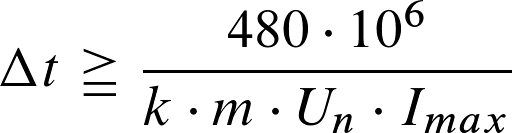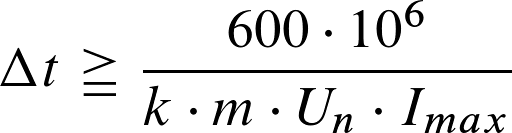# How long does a no-load test take?

Note: The IEC62052-11 edition 2 has introduced a new calculation formula for no-load test. This calculation is valid for meters with type test certificates issued after June 2020.

For all meters before that date the calculation below is valid.

The run-time depends on several factors like

• accuracy class
• meter constant
• number of elements
• Nominal Voltage
• Maximum Current

Test criteria
When the voltage is applied with no current flowing in the current circuit, the test output of the meter shall not produce more than one pulse.
For this test, the current circuit shall be open-circuit and a voltage of 115 % of the reference voltage shall be applied to the voltage circuits.

Minimum Required Time for No-Load Test
nominal voltage V
maximum current A
elements
meter constant i/kWhIEC62053-21 class 2
IEC62053-23 class 2IEC62053-21 class 1
IEC62053-22 class 0.5SIEC62053-22 class 0.2S

For MID meters according to DIN EN BS 50470-3 the calculation formula is different.

Minimum Required Time for No-Load Test EN50470-3
nominal voltage V
nominal current A
elements
meter constant i/kWhDirect connected class A
Direct connected class B
Direct connected class C
CT connected class A
CT connected class B
CT connected class C